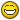Changing line space factor for multiple MtextRecommended PostsHello.

I want to change line space factor for all the mtext objects in a hole bunch of drawings by script.

I found this lisp and it works fine but only by manually selecting the mtext objects.

(setq vla_mtext (vlax-ename->vla-object (setq mymtext (car (entsel)))))

(vla-put-LineSpacingFactor vla_mtext 0.5)

Can this lisp be changed so it changes all mtext objects found in the drawing, or is there another way?

Thanks

Share on other sites(defun C:test ( / acDoc scf cnt )
(setq scf 0.5)
(setq cnt 0)
(vlax-map-collection (vla-get-Block (vla-get-ActiveLayout acDoc))
(function
(lambda (x)
(and
(eq (vla-get-ObjectName x) "AcDbMText")
(eq :vlax-false (vla-get-Lock (vla-item (vla-get-Layers acDoc) (vla-get-Layer x))))
(vlax-property-available-p x 'LineSpacingFactor)
(setq cnt (1+ cnt))
(vla-put-LineSpacingFactor x scf)
)
)
)
)
(if (> cnt 0)
(alert (strcat "\nProceeded " (itoa cnt) " mtext objects! "))
(alert "\nNo mtext objects are found in this tab! ")
)
(princ)

Or maybe just:

(defun C:test ( / scf SS i )
(princ "\nSelect mtexts to change their \"LineSpacingFactor\": ")
(setq scf 0.5)
(if (setq SS (ssget "_:L" (list (cons 0 "MTEXT"))))
(repeat (setq i (sslength SS))
(vla-put-LineSpacingFactor (vlax-ename->vla-object (ssname SS (setq i (1- i)))) scf)
)
)
(if SS (princ (strcat "\nProceeded " (itoa (sslength SS)) " mtext objects! ")))
(princ)

Edited by Grrr
Share on other sitesAnother:

(defun c:mtlsp ( / i s )
(if (setq s (ssget "_X" '((0 . "MTEXT") (-4 . "<>") (44 . 0.5))))
(repeat (setq i (sslength s))
(entmod (append (entget (ssname s (setq i (1- i)))) '((44 . 0.5))))
)
)
(princ)
)

Share on other sitesI can see the next question oh I want a different spacing

(defun c:mtlsp ( / i s rnum)
(setq rnum (getreal "\nEnter spacing <Cr> = 0.5 "))
(if (= rnum nil)(setq rnum 0.5))
(if (setq s (ssget "_X" (list (cons 0 "MTEXT") (cons -4  "<>") (cons 44 rnum))))
(repeat (setq i (sslength s))
(entmod (append (entget (ssname s (setq i (1- i)))) (list (cons 44 rnum))))
)
)
(princ)
)

Share on other sitesI can see the next question oh I want a different spacing

BIGAL - the ProphetShare on other sitesAnother:
(defun c:mtlsp ( / i s )
(if (setq s (ssget "_X" '((0 . "MTEXT") (-4 . "<>") (44 . 0.5))))
(repeat (setq i (sslength s))
(entmod (append (entget (ssname s (setq i (1- i)))) '((44 . 0.5))))
)
)
(princ)
)

This code solved the problem perfectly, thanks again!!!

Share on other sitesYou're most welcomeJoin the conversation

You can post now and register later. If you have an account, sign in now to post with your account.
Note: Your post will require moderator approval before it will be visible.×   Pasted as rich text.   Restore formatting

Only 75 emoji are allowed.